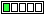All about flooble | fun stuff | Get a free chatterbox | Free JavaScript | Avatarsperplexus dot infoSquare angles (Posted on 2016-08-02)What triangle has all his angles squares (i.e. each angle being represented by a square number of degrees)?

 No Solution Yet Submitted by Ady TZIDON No RatingComments: ( Back to comment list | You must be logged in to post comments.)D1? Full solution. Comment 1 of 1
I didn't do it in my head, but there aren't many numbers to try with the aid of a calculator, thus I consider the problem an easy D2:
180-13^2=11 not the sum of two squares
180-12^2=36 not the sum of two squares
180-11^2=59 not the sum of two squares
180-10^2=80 which is the sum of two squares: 80=8^2+4^2
180-9^2=99 not the sum of two squares

The triangle sought has angles 16, 64, and 100 degrees.

 Posted by Jer on 2016-08-02 12:22:10Please log in:

 Search: Search body:
Forums (0)